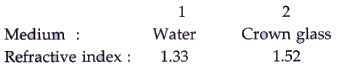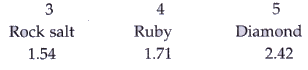Courses

# Solutions of Refraction of Light (Page No- 229 & 239) - Physics By Lakhmir Singh, Class 10 Class 10 Notes | EduRev

## Class 10 : Solutions of Refraction of Light (Page No- 229 & 239) - Physics By Lakhmir Singh, Class 10 Class 10 Notes | EduRev

The document Solutions of Refraction of Light (Page No- 229 & 239) - Physics By Lakhmir Singh, Class 10 Class 10 Notes | EduRev is a part of the Class 10 Course Class 10 Physics Solutions By Lakhmir Singh & Manjit Kaur.
All you need of Class 10 at this link: Class 10

Lakhmir Singh Physics Class 10 Solutions Page No:229

Question 29: The following table gives the refractive indices of a few media :Use this table to give an example of :
(i) a medium pair so that light speeds up when it goes from one of these medium to another.
(ii) a medium pair so that light slows down when it goes from one of these medium to another.

Solution :

(i) Crown glass to water.
(ii) Water to diamond.

Question 30: Refractive indices of four media A, B, C and D are given below :

 Medium Refractive index A 1.33 B 1.44 C 1.52 D 1.65

In which of these four media is the speed of light (i) maximum, and (ii) minimum ?

Solution :

(i) A (It has least refractive index).
(ii) D (It has highest refractive index).

Lakhmir Singh Physics Class 10 Solutions Page No:239

Question 1: Name the lens which can concentrate sun’s rays to a point and burn a hole in a piece of paper.

Solution : Converging lens.

Question 2: Give the usual name for the following :

A point inside a lens through which the light passes undeviated.

Solution : Optical center.

Question 3: A 1 cm high object is placed at a distance of 2ƒ from a convex lens. What is the height of the image formed ?

Solution : 1 cm (same as the height of the object).

Question 4: If the image formed by a convex lens is of the same size as that of the object, what is the position of the image with respect to the lens ?

Solution : At 2F (At twice the focal length).

Question 5: If an object is placed at the focus of a convex lens, where is the image formed ?

Solution : The image is formed at infinity (at very large distance).

Question 6: Where should an object be placed in order to use a convex lens as a magnifying glass ?

Solution : Object should be placed at a distance less than focal length.

Question 7: Where should an object be placed in front of a convex lens so as to obtain its virtual, erect and magnified image ?

Solution : The object should be placed within focus.

Question 8: Where should an object be placed in front of a convex lens so as to obtain its real, inverted and magnified image ?

Solution : Object should be placed between f and 2f.

Offer running on EduRev: Apply code STAYHOME200 to get INR 200 off on our premium plan EduRev Infinity!

94 docs

,

,

,

,

,

,

,

,

,

,

,

,

,

,

,

,

,

,

,

,

,

,

,

,

;